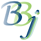#BBjTemplatedString::getFieldAsString

## Description

In BBj 7.0 and higher, this method returns the string value stored in a specified field of a BBjTemplatedString.

## Syntax

Return Value

Method

byte[]

getFieldAsString(string fieldname)

byte[]

getFieldAsString(string fieldname, int index)

## Parameters

Variable

Description

fieldname

The name of the field that is to be retrieved as defined in the template used when constructing the BBjTemplatedString.

index

The index of a repeating field.

## Return Value

Returns the string value that is stored in the BBjTemplatedString.

## Remarks

Methods that return byte[] can usually be treated as if they returned string, because BBj automatically handles the conversion. However, when that byte[] value is directly assigned to an object variable or passed to a Java method, BBj skips the conversion and the raw internal byte[] format is used. You can always use the STR() function to explicitly convert the byte[] value to a string.

## Example

 ``````template\$ = "name:c(32*),context:u(2),code:u(1),id:u(2),objtype:i(2)" dim A\$:template\$ B! = BBjAPI().makeTemplatedString(template\$) ?"template for A\$: ", FATTR(A\$) ?"template for B!: ", B!.fattr() A.name\$="aaa" A.name\$ = "eee" A.context = 22 A.code = 3 A.id = 44 A.objType = 55 B!.setString(A\$) ?B!.getFieldAsString("name",1)," = ", A.name\$ ?B!.getFieldAsString("name",5)," = ", A.name\$ ?B!.getFieldAsNumber("context")," = ", A.context ?B!.getFieldAsNumber("code"), " = ", A.code ?B!.getFieldAsNumber("id"), " = ", A.id ?B!.getFieldAsNumber("objType")," = ", A.objType B!.setFieldValue("name",1,"XXX") B!.setFieldValue("name",5,"ZZZ") B!.setFieldValue("context",66) B!.setFieldValue("code",7) B!.setFieldValue("id",88) B!.setFieldValue("objType",9) A\$ = B!.getString() ? ?B!.getFieldAsString("name",1)," = ", A.name\$ ?B!.getFieldAsString("name",5)," = ", A.name\$ ?B!.getFieldAsNumber("context")," = ", A.context ?B!.getFieldAsNumber("code"), " = ", A.code ?B!.getFieldAsNumber("id"), " = ", A.id ?B!.getFieldAsNumber("objType")," = ", A.objType``````